# MATLAB中histogram函数的使用

histogram函数的作用是绘制直方图

直方图属于数值数据的条形图类型，将数据分组为 bin。创建 Histogram 对象后，可以通过更改直方图的属性值修改它的各个方面。这对快速修改 bin 属性或更改显示特别有用。

## 语法

``````histogram(X)
histogram(X,nbins)
histogram(X,edges)
histogram('BinEdges',edges,'BinCounts',counts)
histogram(C)
histogram(C,Categories)
histogram('Categories',Categories,'BinCounts',counts)
histogram(___,Name,Value)
histogram(ax,___)
h = histogram(___)``````

## 说明

​histogram(X) 基于 X 创建直方图。histogram 函数使用自动分 bin 算法，然后返回均匀宽度的 bin，这些 bin 可涵盖 X 中的元素范围并显示分布的基本形状。histogram 将 bin 显示为矩形，这样每个矩形的高度就表示 bin 中的元素数量。​

​histogram(X,nbins) 使用标量 nbins 指定的 bin 数量。

​histogram(X,edges) 将 X 划分为由向量 edges 来指定 bin 边界的 bin。每个 bin 都包含左边界，但不包含右边界，除了同时包含两个边界的最后一个 bin 外。

histogram('BinEdges',edges,'BinCounts',counts) 手动指定 bin 边界和关联的 bin 计数。histogram 绘制指定的 bin 计数，而不执行任何数据分 bin。

​histogram(C)（其中 C 为分类数组）通过为 C 中的每个类别绘制一个条形来绘制直方图。

histogram(C,Categories) 仅绘制 Categories 指定的类别的子集。

histogram('Categories',Categories,'BinCounts',counts) 手动指定类别和关联的 bin 计数。histogram 绘制指定的 bin 计数，而不执行任何数据分 bin。

​histogram(___,Name,Value) 使用前面的任何语法指定具有一个或多个 Name,Value 对组参数的其他选项。例如，可以指定 'BinWidth' 和一个标量以调整 bin 的宽度，或指定 'Normalization' 和一个有效选项（'count'、'probability'、'countdensity'、'pdf'、'cumcount' 或 'cdf'）以使用不同类型的归一化。

histogram(ax,___) 将图形绘制到 ax 指定的坐标区中，而不是当前坐标区 (gca) 中。选项 ax 可以位于前面的语法中的任何输入参数组合之前。

h = histogram(___) 返回 Histogram 对象。使用此语法可检查并调整直方图的属性。

## 示例

### 向量直方图​

​生成 10,000 个随机数并创建直方图。histogram 函数自动选择合适的 bin 数量，以便涵盖 x 中的值范围并显示基本分布的形状。

``````x = randn(10000,1);
h = histogram(x)
``````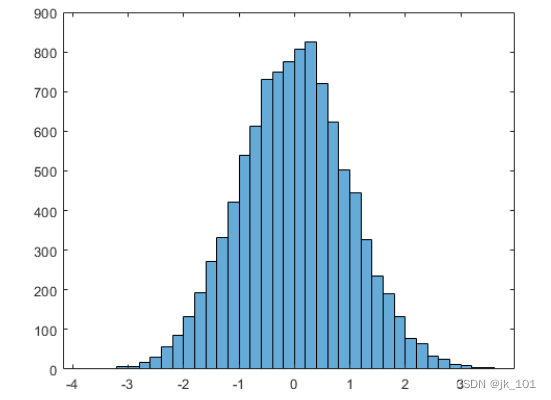``````h =
Histogram with properties:

Data: [10000x1 double]
Values: [2 2 1 6 7 17 29 57 86 133 193 271 331 421 540 613 ... ]
NumBins: 37
BinEdges: [-3.8000 -3.6000 -3.4000 -3.2000 -3 -2.8000 -2.6000 ... ]
BinWidth: 0.2000
BinLimits: [-3.8000 3.6000]
Normalization: 'count'
FaceColor: 'auto'
EdgeColor: [0 0 0]

Show all properties``````

指定 histogram 函数的输出参数时，它返回一个二元直方图对象。可以使用该对象检查直方图的属性，例如 bin 数量或宽度。

计算直方图的 bin 数量。

``````nbins = h.NumBins

nbins = 37``````

### 指定直方图的 bin 数量

对分类为 25 个等距 bin 的 1,000 个随机数绘制直方图。

``````x = randn(1000,1);
nbins = 25;
h = histogram(x,nbins)``````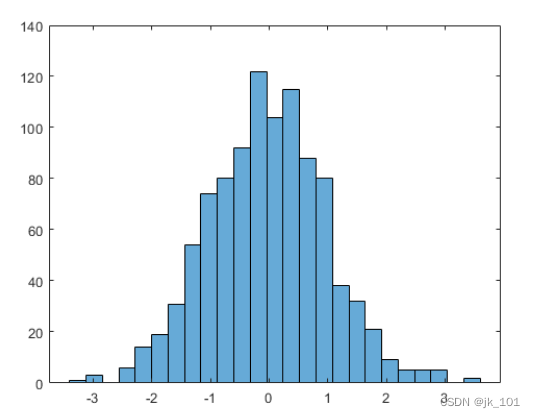``````h =
Histogram with properties:

Data: [1000x1 double]
Values: [1 3 0 6 14 19 31 54 74 80 92 122 104 115 88 80 38 32 ... ]
NumBins: 25
BinEdges: [-3.4000 -3.1200 -2.8400 -2.5600 -2.2800 -2 -1.7200 ... ]
BinWidth: 0.2800
BinLimits: [-3.4000 3.6000]
Normalization: 'count'
FaceColor: 'auto'
EdgeColor: [0 0 0]

Show all properties
``````

求 bin 计数。

``````counts = h.Values

counts = 1×25

1     3     0     6    14    19    31    54    74    80    92   122   104   115    88    80    38    32    21     9     5     5     5     0     2
``````

### 更改直方图的 bin 数量

生成 1,000 个随机数并创建直方图。

``````X = randn(1000,1);
h = histogram(X)``````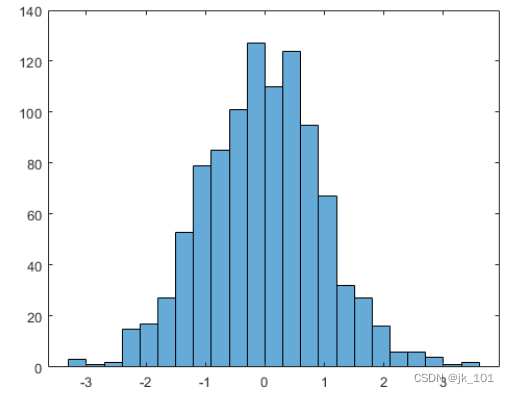``````h =
Histogram with properties:

Data: [1000x1 double]
Values: [3 1 2 15 17 27 53 79 85 101 127 110 124 95 67 32 27 ... ]
NumBins: 23
BinEdges: [-3.3000 -3.0000 -2.7000 -2.4000 -2.1000 -1.8000 ... ]
BinWidth: 0.3000
BinLimits: [-3.3000 3.6000]
Normalization: 'count'
FaceColor: 'auto'
EdgeColor: [0 0 0]

Show all properties``````

使用 morebins 函数粗略调整 bin 数量。

``````Nbins = morebins(h);
Nbins = morebins(h)``````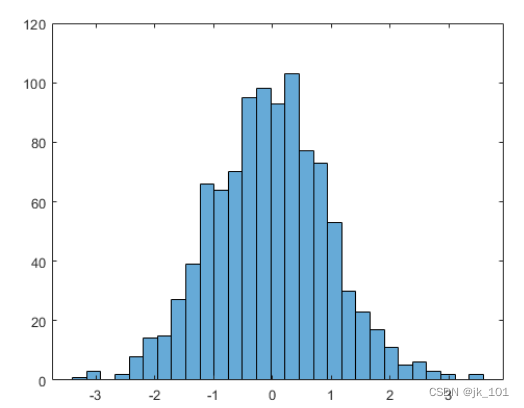``Nbins = 29``

通过显式设置 bin 数按精细颗粒级别调整 bin。

``h.NumBins = 31;``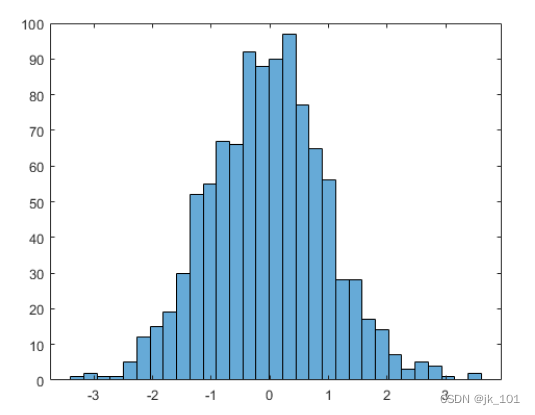### 指定直方图的 bin 边界

生成 1,000 个随机数并创建直方图。将 bin 边界指定为向量，使宽 bin 在直方图的两边，以捕获不满足 ∣x∣<2 的离群值。第一个向量元素是第一个 bin 的左边界，而最后一个向量元素是最后一个 bin 的右边界。

``````x = randn(1000,1);
edges = [-10 -2:0.25:2 10];
h = histogram(x,edges);``````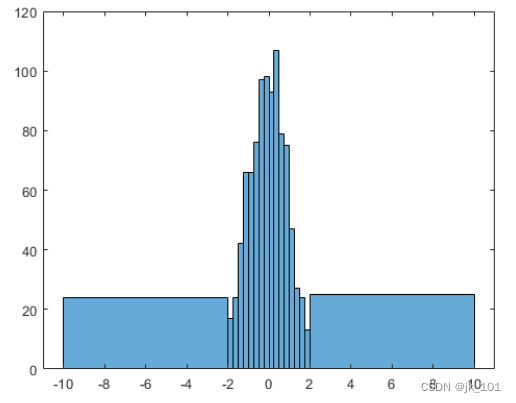将 Normalization 属性指定为 'countdensity' 以使包含离群值的 bin 扁平化。现在，每个 bin 的区域（而不是高度）表示该 bin 的观测值频率。

``h.Normalization = 'countdensity';``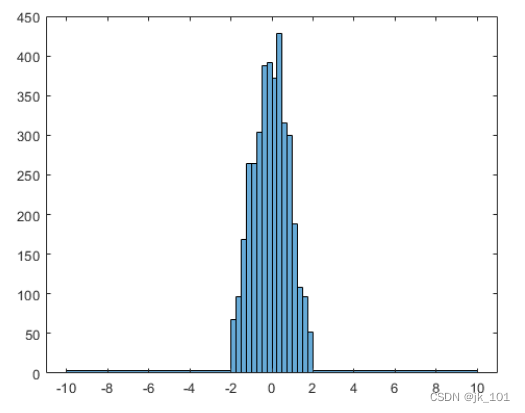### 绘制分类直方图

创建一个表示投票的分类向量。该向量中的类别是 'yes'、'no' 或 'undecided'。

``````A = [0 0 1 1 1 0 0 0 0 NaN NaN 1 0 0 0 1 0 1 0 1 0 0 0 1 1 1 1];
C = categorical(A,[1 0 NaN],{'yes','no','undecided'})
C = 1x27 categorical
Columns 1 through 9

no      no      yes      yes      yes      no      no      no      no

Columns 10 through 16

undecided      undecided      yes      no      no      no      yes

Columns 17 through 25

no      yes      no      yes      no      no      no      yes      yes

Columns 26 through 27

yes      yes ``````

使用相对条形宽度 `0.5` 绘制投票的分类直方图。

``h = histogram(C,'BarWidth',0.5)``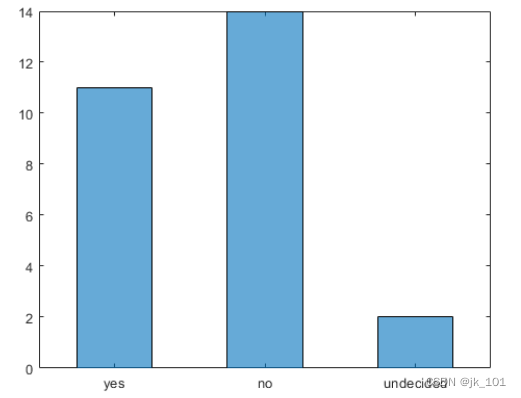``````h =
Histogram with properties:

Data: [no    no    yes    yes    yes    no    no    ...    ]
Values: [11 14 2]
NumDisplayBins: 3
Categories: {'yes'  'no'  'undecided'}
DisplayOrder: 'data'
Normalization: 'count'
DisplayStyle: 'bar'
FaceColor: 'auto'
EdgeColor: [0 0 0]

Show all properties``````

### 具有指定归一化的直方图

生成 1,000 个随机数并使用 'probability' 归一化创建直方图。

``````x = randn(1000,1);
h = histogram(x,'Normalization','probability')``````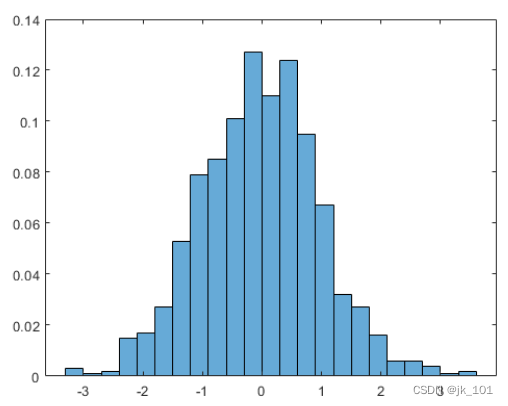``````h =
Histogram with properties:

Data: [1000x1 double]
Values: [0.0030 1.0000e-03 0.0020 0.0150 0.0170 0.0270 0.0530 ... ]
NumBins: 23
BinEdges: [-3.3000 -3.0000 -2.7000 -2.4000 -2.1000 -1.8000 ... ]
BinWidth: 0.3000
BinLimits: [-3.3000 3.6000]
Normalization: 'probability'
FaceColor: 'auto'
EdgeColor: [0 0 0]

Show all properties``````

计算条形高度的总和。通过该归一化，每个条形的高度等于在该 bin 间隔内选择观测值的概率，并且所有条形的高度总和为 1。

``````S = sum(h.Values)

S = 1``````

### 绘制多个直方图

生成两个随机数向量并在同一图窗中针对每个向量绘制对应的一个直方图。

``````x = randn(2000,1);
y = 1 + randn(5000,1);
h1 = histogram(x);
hold on
h2 = histogram(y);``````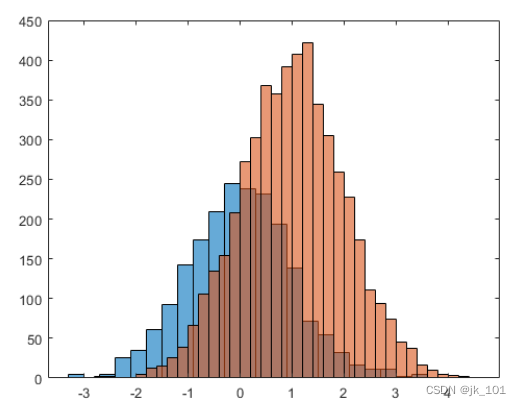由于直方图的示例大小和 bin 宽度不同，很难将它们进行比较。对这些直方图进行归一化，这样所有的条形高度相加的结果为 1 并使用统一的 bin 宽度。

``````h1.Normalization = 'probability';
h1.BinWidth = 0.25;
h2.Normalization = 'probability';
h2.BinWidth = 0.25;``````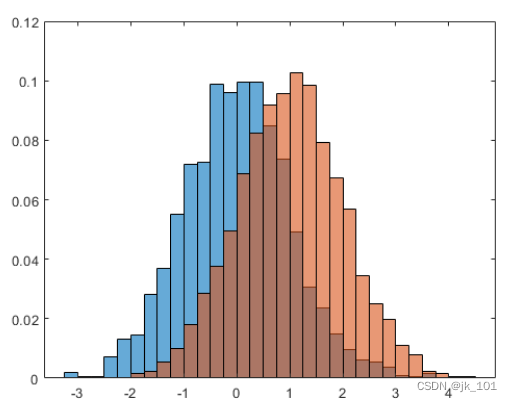### 调整直方图属性

生成 1,000 个随机数并创建直方图。返回直方图对象以调整该直方图的属性，无需重新创建整个绘图。

``````x = randn(1000,1);
h = histogram(x)``````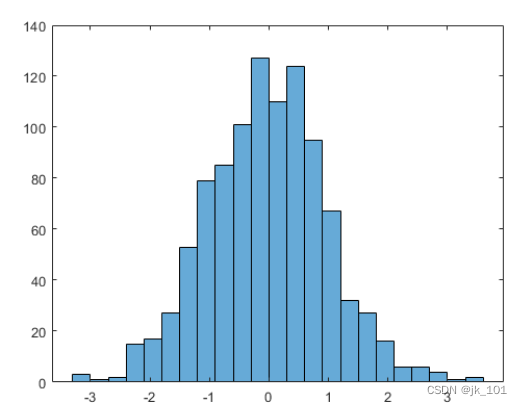``````h =
Histogram with properties:

Data: [1000x1 double]
Values: [3 1 2 15 17 27 53 79 85 101 127 110 124 95 67 32 27 ... ]
NumBins: 23
BinEdges: [-3.3000 -3.0000 -2.7000 -2.4000 -2.1000 -1.8000 ... ]
BinWidth: 0.3000
BinLimits: [-3.3000 3.6000]
Normalization: 'count'
FaceColor: 'auto'
EdgeColor: [0 0 0]

Show all properties
``````

准确指定要使用的 bin 数量。

``h.NumBins = 15;``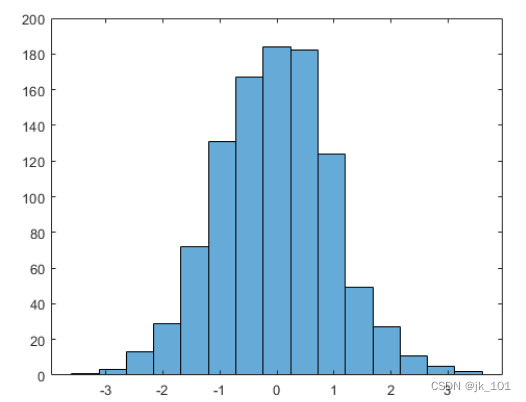通过向量指定 bin 边界。向量中的第一个值是第一个 bin 的左边界。最后一个值是最后一个 bin 的右边界。

``h.BinEdges = [-3:3];``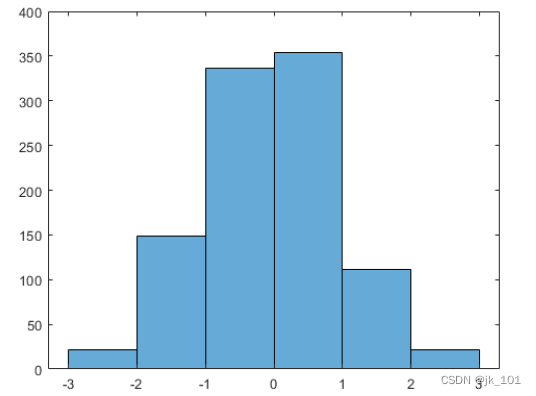更改直方图条形的颜色。

``````h.FaceColor = [0 0.5 0.5];
h.EdgeColor = 'r';``````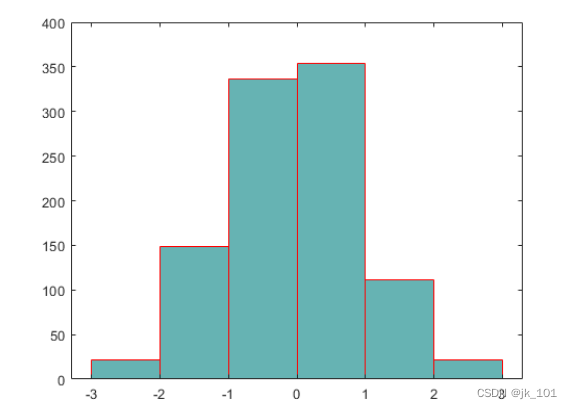### 确定基本概率分布

生成 5,000 个均值为 5、标准差为 2 的正态分布随机数。在 `Normalization` 设为 `'pdf'` 的情况下绘制直方图可生成概率密度函数的估计值。

``````x = 2*randn(5000,1) + 5;
histogram(x,'Normalization','pdf')``````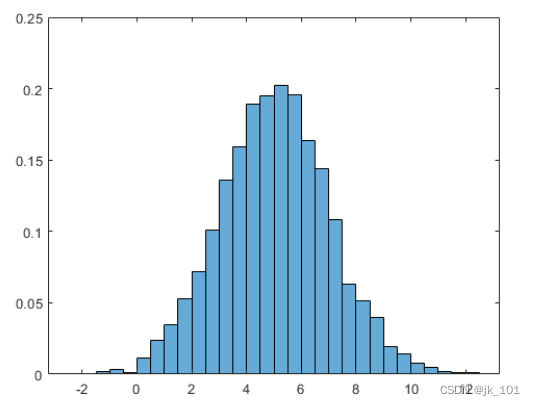在本示例中，已知正态分布数据的基本分布。但是，通过将它与已知的概率密度函数进行对比，可以使用 `'pdf'` 直方图确定该数据的基础概率分布。

均值为 μ、标准差为 σ 以及方差为 σ^2 的正态分布的概率密度函数是：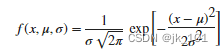对于均值为 5、标准差为 2 的正态分布，叠加一个概率密度函数图。

``````hold on
y = -5:0.1:15;
mu = 5;
sigma = 2;
f = exp(-(y-mu).^2./(2*sigma^2))./(sigma*sqrt(2*pi));
plot(y,f,'LineWidth',1.5)``````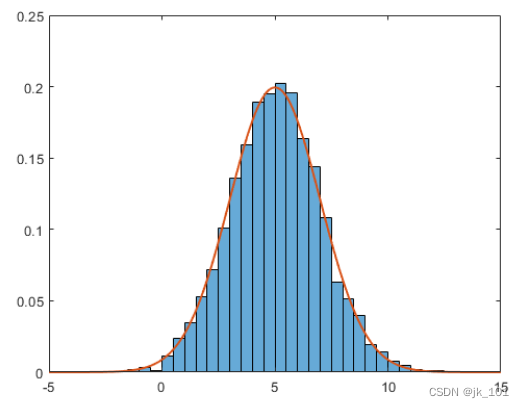### 保存并加载直方图对象

使用 savefig 函数保存 histogram 图窗。

``````histogram(randn(10));
savefig('histogram.fig');
close gcf``````

使用 openfig 重新将直方图加载到 MATLAB。openfig 也返回图窗 h 的句柄。

``h = openfig('histogram.fig');``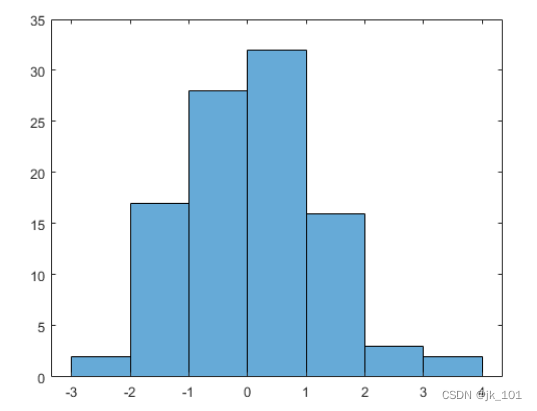使用 findobj 函数从图窗句柄中查找正确的对象句柄。这样，您可以继续处理用于生成图窗的原始直方图对象。

``````y = findobj(h,'type','histogram')

y =
Histogram with properties:

Data: [10x10 double]
Values: [2 17 28 32 16 3 2]
NumBins: 7
BinEdges: [-3 -2 -1 0 1 2 3 4]
BinWidth: 1
BinLimits: [-3 4]
Normalization: 'count'
FaceColor: 'auto'
EdgeColor: [0 0 0]

Show all properties
``````

• 使用 histogram 创建的直方图在绘图编辑模式下提供上下文菜单，以允许在图窗窗口中进行交互式操作。例如，您可以使用上下文菜单以交互方式更改 bin 的数量、对齐多个直方图或更改显示顺序。

• 当向直方图添加数据提示时，它们会显示 bin 边界和 bin 计数。

• 来源：jk_101